# Introduction to NengoSPA¶

This tutorial introduces the usage of NengoSPA. It expects some basic familarity with Nengo. If you have used the legacy SPA implementation shipped with core Nengo, you might want to read this alternate introduction.

We recommend to import nengo_spa as spa. (Note that this uses an underscore in the module name and is different from nengo.spa which refers to the legacy SPA module shipped with core Nengo.)

:

from __future__ import print_function

%matplotlib inline

import nengo
import numpy as np
import matplotlib.pyplot as plt

import nengo_spa as spa

seed = 0
rng = np.random.RandomState(seed + 1)


We will have to specify the dimensionality of the Semantic Pointers. To make it easy to change in all places, we define the variable d here and set it to 32. A dimensionality of 32 is on the lower end (in most actual models you will want to use at least 64 dimensions and we have been using up to 512 dimensions), but it makes the examples in this introduction run faster.

:

d = 32


## Hello world¶

Let us start with a very simple model to demonstrate the basic usage of NengoSPA:

:

with spa.Network(seed=seed) as model:
stimulus = spa.Transcode("Hello", output_vocab=d)
state = spa.State(vocab=d)
nengo.Connection(stimulus.output, state.input)
p = nengo.Probe(state.output, synapse=0.01)


The first thing to notice is that instead of nengo.Network, we use spa.Network here. This allows use to more easily use Nengo’s config system for NengoSPA (something we will look at in more detail later). Then we instantiate two networks spa.Transcode and spa.State. These networks are aware of Semantic Pointer inputs and/or outputs. Such networks we also call (SPA) modules. The Transcode module is similar to a nengo.Node. Here it is given a the constant Semantic Pointer Hello and it will output this pointer during the whole simulation. The State module is a network of Nengo ensembles that is optimized for representing (unit-length) Semantic Pointers. Both of these modules have a vocab-like argument which is short for vocabulary. In the context of NengoSPA a vocabulary is a set of Semantic Pointers with a certain dimensionality. Here we just use the default vocabulary with dimensionality d. The required Semantic Pointers (in this example Hello) will be automatically added to that vocabulary.

Modules can be used like normal Nengo networks. Thus, we can create a connection from the output of stimulus to the input of state and then probe the output of state.

:

with nengo.Simulator(model) as sim:
sim.run(0.5)


Now, let us plot the probed data:

:

plt.plot(sim.trange(), sim.data[p])
plt.xlabel("Time [s]")

:

Text(0.5, 0, 'Time [s]')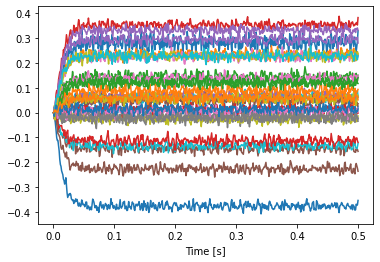This plot displays the raw vector components of the represented Semantic Pointer and is not extremely helpful. A useful function to get a more informative plot is spa.similarity. It takes the probe data and a vocabulary as arguments, and returns the similarity of the data to each Semantic Pointer in the vocabulary. We can access the vocabulary with the vocab attribute of the State module.

:

plt.plot(sim.trange(), spa.similarity(sim.data[p], state.vocab))
plt.xlabel("Time [s]")
plt.ylabel("Similarity")
plt.legend(state.vocab, loc="best")

:

<matplotlib.legend.Legend at 0x7f107eda6a90>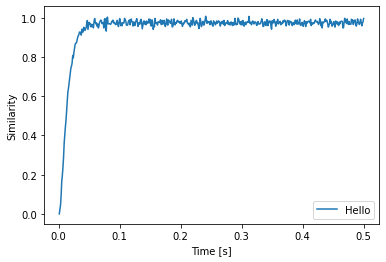We see that input Pointer is successfully represented.

## SPA syntax¶

One of the main features of nengo_spa is a special syntax that makes it easier to construct large SPA models. Let us demonstrate this with a slightly more complicated example where we take represent a scene by a Semantic Pointer BLUE * CIRCLE + RED * SQUARE (* denotes circular convolution here) and use another input to selectively retrieve the color of one of the objects.

:

with spa.Network(seed=seed) as model:
scene = spa.Transcode("BLUE * CIRCLE + RED * SQUARE", output_vocab=d)
query = spa.Transcode(lambda t: "CIRCLE" if t < 0.25 else "SQUARE", output_vocab=d)
result = spa.State(vocab=d)

scene * ~query >> result

p = nengo.Probe(result.output, synapse=0.01)


We see that the Transcode object also accepts functions like a Nengo Node. But the really interesting line is:

scene * ~query >> result


This line is all that is needed to construct a network inverting a circular convolution (* is the circular convolution, ~ inverts query), connect scene and query as inputs, and then connect the output to result (>>).

If we run the model, we see that is successfully decodes BLUE and RED.

:

with nengo.Simulator(model) as sim:
sim.run(0.5)

:

plt.plot(sim.trange(), spa.similarity(sim.data[p], result.vocab))
plt.xlabel("Time [s]")
plt.ylabel("Similarity")
plt.legend(result.vocab, loc="best")

:

<matplotlib.legend.Legend at 0x7f107ef21400>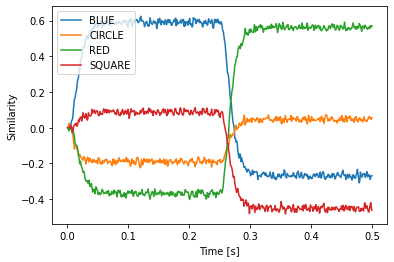Besides circular convolution (*) and the approximate inverse (~), you can use * also to scale with vectors with a fixed scalar or multiply to scalars, + and - to add and subtract Semantic Pointers, and @ to compute a dot product. To compute a dot product with older python versions use the spa.dot function.

### Symbols¶

So far we used the Transcode network to provide fixed inputs to the network. But there is a second method that sometimes means less typing.

:

with spa.Network(seed=seed) as model:
query = spa.Transcode(lambda t: "CIRCLE" if t < 0.25 else "SQUARE", output_vocab=d)
result = spa.State(vocab=d)

((spa.sym.BLUE * spa.sym.CIRCLE + spa.sym.RED * spa.sym.SQUARE) * ~query >> result)

p = nengo.Probe(result.output, synapse=0.01)


The spa.sym object is a special object on which you can access arbitrary attributes that will magically return symbolic representations of Semantic Pointers that can be combined with the usual operators among each other or with SPA modules which will cause the required neural networks to be implemented.

spa.sym can also be called as a function:

:

with spa.Network(seed=seed) as model:
query = spa.Transcode(lambda t: "CIRCLE" if t < 0.25 else "SQUARE", output_vocab=d)
result = spa.State(vocab=d)

spa.sym("BLUE * CIRCLE + RED * SQUARE") * ~query >> result

p = nengo.Probe(result.output, synapse=0.01)


### Action selection¶

Often it is necessary to choose one action out of a number of potential actions. This can be done with the ActionSelection object. It is used as a context manager und within its context ifmax function calls define the potential actions. You can pass an optional name as first argument to ifmax, but you don’t have to. The next argument is an expression that determines the utility value for the action being defined. The remaining arguments define where things should be routed, if the action’s utility value is the highest of all the actions defined in the ActionSelection context.

In this example, we use this to cycle through a sequence of Semantic Pointers. Initially, we feed A into state to start things off and then we compute the dot product of the Semantic Pointer in state with the candidates and feed the next Semantic Pointer in the sequence to state accordingly.

:

def start(t):
if t < 0.05:
return "A"
else:
return "0"

with spa.Network(seed=seed) as model:
state = spa.State(d)
spa_input = spa.Transcode(start, output_vocab=d)

spa_input >> state
with spa.ActionSelection():
spa.ifmax(spa.dot(state, spa.sym.A), spa.sym.B >> state)
spa.ifmax(spa.dot(state, spa.sym.B), spa.sym.C >> state)
spa.ifmax(spa.dot(state, spa.sym.C), spa.sym.D >> state)
spa.ifmax(spa.dot(state, spa.sym.D), spa.sym.E >> state)
spa.ifmax(spa.dot(state, spa.sym.E), spa.sym.A >> state)

p = nengo.Probe(state.output, synapse=0.01)

:

with nengo.Simulator(model) as sim:
sim.run(0.5)

:

plt.plot(sim.trange(), spa.similarity(sim.data[p], state.vocab))
plt.legend(state.vocab.keys())
plt.xlabel("Time [s]")
plt.ylabel("Similarity")

:

Text(0, 0.5, 'Similarity')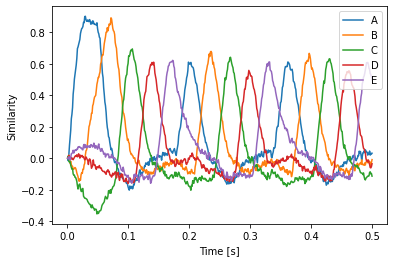## Using Transcode for computation¶

As mentioned before, the Transcode object is the analog to the Nengo Node. That means you can also use it for computation implemented in Python code. Let us take one of the previous examples, but implement the approximate inverse of the circular convolution in Python code. Note that scene and x are both SPA symbols, so the operations * and ~ still apply as defined in the SPA syntax.

:

with spa.Network(seed=seed) as model:
scene = spa.sym.BLUE * spa.sym.CIRCLE + spa.sym.RED * spa.sym.SQUARE
unbind = spa.Transcode(lambda t, x: scene * ~x, input_vocab=d, output_vocab=d)
query = spa.Transcode(lambda t: "CIRCLE" if t < 0.25 else "SQUARE", output_vocab=d)
result = spa.State(vocab=d)

query >> unbind
unbind >> result

p = nengo.Probe(result.output, synapse=0.01)

:

with nengo.Simulator(model) as sim:
sim.run(0.5)

:

plt.plot(sim.trange(), spa.similarity(sim.data[p], result.vocab))
plt.xlabel("Time [s]")
plt.ylabel("Similarity")
plt.legend(result.vocab, loc="best")

:

<matplotlib.legend.Legend at 0x7f1075e846d0>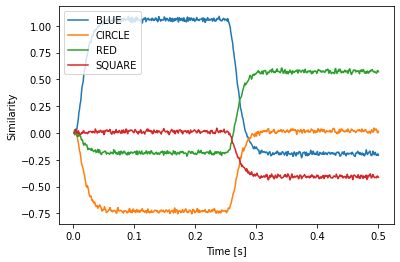## Using the config system with NengoSPA¶

When building networks with NengoSPA there is a number of parameters that will be set to predetermined values. Sometimes you might want to change these values. That can be done with the Nengo config system. In this example, we change the number of neurons implementing a State. Because we reduce the number of neurons by a lot, the start of the result will look much noisier.

:

with spa.Network(seed=seed) as model:
model.config[spa.State].neurons_per_dimension = 10

state = spa.State(d)
spa.sym.A >> state

p = nengo.Probe(state.output, synapse=0.01)

:

with nengo.Simulator(model) as sim:
sim.run(0.5)

:

plt.plot(sim.trange(), spa.similarity(sim.data[p], state.vocab))
plt.legend(state.vocab.keys())
plt.xlabel("Time [s]")
plt.ylabel("Similarity")

:

Text(0, 0.5, 'Similarity')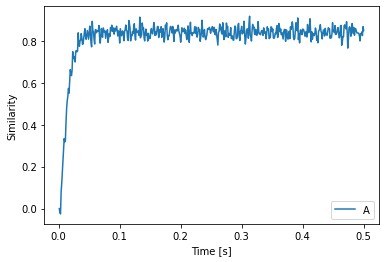## Vocabularies¶

Sometimes you will have sets of unrelated Semantic Pointers. It can be useful to keep them in distinct groups called vocabularies. You can create a vocabulary by instantiating a spa.Vocabulary object with the desired dimensionality. To fill it with Semantic Pointers, you can use the populate method which takes a string of Semantic Pointer names separated with semicolons. The vocabulary object will try to keep the maximum similarity between all pointers below a certain threshold (0.1 by default).

:

vocab = spa.Vocabulary(32, pointer_gen=rng)
vocab.populate("BLUE; RED; CIRCLE; SQUARE")


If you happen to have a Python list of names, that can also be passed easily to populate with a little trick:

:

list_of_names = ["GREEN", "YELLOW"]
vocab.populate(";".join(list_of_names))


You can also construct Semantic Pointers out of others.

:

vocab.populate(
"""
PURPLE = BLUE + RED;
COLOR_MIX_1 = 0.8 * GREEN + 0.5 * YELLOW;
SQUARING_THE_CIRCLE = CIRCLE * SQUARE"""
)


Note that these Semantic Pointers will not be normalized to unit length. If you desire so, call normalized() on the constructed pointer. You can also use unitary() to get unitary Semantic Pointers.

:

vocab.populate(
"""
NORM_PURPLE = (BLUE + RED).normalized();
UNITARY1.unitary();
UNITARY2 = (SQUARE * CIRCLE).unitary()"""
)


Sometimes you might already have a vector that you want to use as a Semantic Pointer instead of a randomly constructed one. These can be added to a vocabulary with the add method. Note, that this will not ensure the maximum similarity constraint.

:

v = np.zeros(32)


Vocabularies work like dictionaries. That means you can retrieve all contained Semantic Pointers with keys() and use indexing with Semantic Pointer names to retrieve specific pointers as SemanticPointer objects. Those specific pointers support the associated arithmetic operations (e.g., + for superposition, * for circular convolution). They can also be used in the SPA syntax, for example to provide them as input to modules. To access the actual vector use the v attribute.

:

print(list(vocab.keys()))

['BLUE', 'RED', 'CIRCLE', 'SQUARE', 'GREEN', 'YELLOW', 'PURPLE', 'COLOR_MIX_1', 'SQUARING_THE_CIRCLE', 'NORM_PURPLE', 'UNITARY1', 'UNITARY2', 'NULL']

:

blue = vocab["BLUE"]
print(blue.v)
print((blue * vocab["RED"]).v)

[ 0.29037237 -0.10935923 -0.09441741 -0.19180677  0.15470261 -0.41142928
0.31190727 -0.1360754   0.05703229 -0.04457812  0.26137037 -0.36827633
-0.05763617 -0.0686546   0.20267569 -0.19661954 -0.03082373 -0.15692835
0.00754624  0.10418562 -0.19674966  0.20463391  0.1611708   0.08982725
0.16103945 -0.12222504 -0.02196819 -0.16728068 -0.0478884   0.09480778
-0.12364315 -0.07092473]
[-2.33592906e-01  1.20230697e-01 -1.81461860e-01  1.51189322e-01
-3.02394960e-02  1.80646918e-01  2.04080856e-02  2.67529926e-01
-1.71525203e-01 -1.49076698e-01 -3.89136163e-01  7.70771473e-02
1.03376788e-02 -1.84943800e-02 -2.78839585e-02  3.48011970e-01
4.95260398e-02  2.66837761e-02 -1.65875900e-01  5.28879043e-02
-1.97860208e-01  3.28975664e-01 -3.15501481e-01 -1.57759818e-01
6.05768126e-03  2.02859688e-01 -3.12011730e-01  7.45078372e-02
-1.87441936e-01  2.31766730e-01 -5.49585899e-02  2.16370278e-04]


A vocabulary can also parse more complex expressions and return the resulting Semantic Pointer.

:

print(vocab.parse("BLUE * RED").v)

[-2.33592906e-01  1.20230697e-01 -1.81461860e-01  1.51189322e-01
-3.02394960e-02  1.80646918e-01  2.04080856e-02  2.67529926e-01
-1.71525203e-01 -1.49076698e-01 -3.89136163e-01  7.70771473e-02
1.03376788e-02 -1.84943800e-02 -2.78839585e-02  3.48011970e-01
4.95260398e-02  2.66837761e-02 -1.65875900e-01  5.28879043e-02
-1.97860208e-01  3.28975664e-01 -3.15501481e-01 -1.57759818e-01
6.05768126e-03  2.02859688e-01 -3.12011730e-01  7.45078372e-02
-1.87441936e-01  2.31766730e-01 -5.49585899e-02  2.16370278e-04]


### Creating subsets of vocabularies¶

In some cases it is useful to create a smaller subset from an existing vocabulary. This subset will use exactly the same vectors as in the original vocabulary.

:

just_colors = vocab.create_subset(["BLUE", "RED", "GREEN", "YELLOW", "PURPLE"])
print(list(just_colors.keys()))

['BLUE', 'RED', 'GREEN', 'YELLOW', 'PURPLE']


### Using vocabularies¶

To use a manually created vocabulary in a model, pass it instead of the dimensionality to the module.

:

with spa.Network() as model:
state = spa.State(vocab)


### Strict mode¶

Vocabularies that are manually instantiated will be in strict mode per default. That means trying to access a Semantic Pointer that does not exist in the vocabulary gives an exception. That can sometimes catch spelling mistakes.

:

try:
vocab.parse("UNKNOWN")
except spa.exceptions.SpaParseError as err:
print(err)

Error parsing expression 'UNKNOWN' with 32-dimensional vocab  at 0x7f1075aa9f70: name 'UNKNOWN' is not defined


In some cases, however, it is more useful to have unknown Semantic Pointers added to the vocabulary automatically. This happens when a vocabulary is created in non-strict mode.

:

non_strict_vocab = spa.Vocabulary(32, strict=False)
non_strict_vocab.parse("UNKNOWN")

:

SemanticPointer(array([ 0.07913094,  0.10078531,  0.13879982,  0.29690737, -0.19026915,
-0.22988261,  0.00715086,  0.02121388,  0.05514268,  0.08256244,
-0.14690477,  0.32214103, -0.07238899,  0.15708512,  0.21678787,
0.15400449, -0.29093543, -0.36521131,  0.21396055,  0.19715409,
0.04109584,  0.23113396,  0.12493312, -0.03672708, -0.18588779,
-0.0174775 ,  0.23159449,  0.00839069,  0.00560407,  0.29511521,
-0.00684496, -0.01704141]), vocab=<nengo_spa.vocabulary.Vocabulary object at 0x7f1075aa2070>, algebra=<nengo_spa.algebras.hrr_algebra.HrrAlgebra object at 0x7f1080fb66a0>, name='UNKNOWN'


The default vocabularies in NengoSPA that are being used whenever you just specify the dimensionality for a module will be in non-strict mode.

### Converting between vocabularies¶

When you want to combine Semantic Pointers from different vocabularies in any way, you will need to convert the Semantic Pointers from one vocabulary to the other vocabulary. There are different methods to do so.

First, you can use reinterpret to indicate that Semantic Pointers from one vocabulary should be interpreted in the context of another vocabulary without any change the actual represented vector. That requires both vocabularies to have the same dimensionality.

:

with spa.Network() as model:
color = spa.State(just_colors)
state = spa.State(vocab)
spa.reinterpret(color, vocab) >> state


Second, you can use ‘translate’ to find a linear transform that tries to project the Semantic Pointers from one vocabulary to Semantic Pointers in the other vocabulary based on the names. This can be useful to use different dimensionalities for the same Semantic Pointers in different parts of your model.

:

pointers = "ONE; TWO; THREE"

low_d = spa.Vocabulary(32)
low_d.populate(pointers)

high_d = spa.Vocabulary(128)
high_d.populate(pointers)

:

with spa.Network() as model:
low_d_state = spa.State(low_d)
high_d_state = spa.State(high_d)
spa.translate(low_d_state, high_d) >> high_d_state


## Clean up memories¶

The unbinding of a vector is only an approximate inverse. That means the result will contain some noise that in some cases needs to be removed. This can be done with clean-up or associative memories (a clean-up memory is basically an auto-associative memory). NengoSPA provides a number of different variants of such associative memories. Here we demonstrate the clean-up with a ThresholdingAssocMem. It simply removes all vector components below a certain threshold (here 0.2).

An associative memory needs to be provided with a vocabulary and all potential Semantic Pointers that it could clean up to with the mapping parameter. The mapping parameters allows to specify hetero-associative mappings, but here we just provide a sequence of keys which will create an auto-associative mapping.

Note that this subset is considered to be a different vocabulary than the default vocabulary itself. That means a simple result >> am will not work. But we know that both vocabularies use exactly the same vectors (because we created one as subset from the other) and thus can reinterpret the Semantic Pointer from result in the subset vocabulary. This is done with spa.reinterpret(result) >> am. In this case we do not need to provide the vocabulary as argument to spa.reinterpret because it can be determined from the context. Keep in mind that this might not work in all cases and give an exception. Then you have to explicitly add the vocabulary as argument to spa.reinterpret.

:

with spa.Network(seed=seed) as model:
scene = spa.Transcode("BLUE * CIRCLE + RED * SQUARE", output_vocab=d)
query = spa.Transcode(lambda t: "CIRCLE" if t < 0.25 else "SQUARE", output_vocab=d)
result = spa.State(d)
am = spa.ThresholdingAssocMem(0.2, input_vocab=d, mapping=["BLUE", "RED"])

scene * ~query >> result
spa.reinterpret(result) >> am

p_result = nengo.Probe(result.output, synapse=0.01)
p_am = nengo.Probe(am.output, synapse=0.01)

:

with nengo.Simulator(model) as sim:
sim.run(0.5)

:

plt.subplot(1, 2, 1)
plt.plot(sim.trange(), spa.similarity(sim.data[p_result], model.vocabs[d]))
plt.title("Before clean-up")
plt.xlabel("Time [s]")
plt.ylabel("Similarity")
plt.legend(model.vocabs[d], loc="lower left")

plt.subplot(1, 2, 2, sharey=plt.gca())
plt.plot(sim.trange(), spa.similarity(sim.data[p_am], model.vocabs[d]))
plt.title("After clean-up")
plt.xlabel("Times [s]")

:

Text(0.5, 0, 'Times [s]')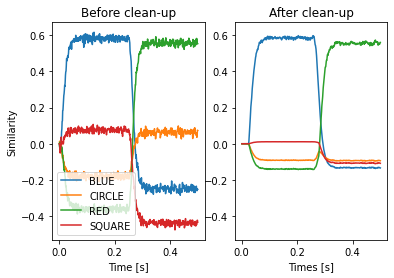## What is next?¶

This introduction gave a short overview of the core NengoSPA features. But there is a lot more to most of them. Thus, you might want to delve deeper into certain parts of the documentation that are relevant to you or look at specific examples.